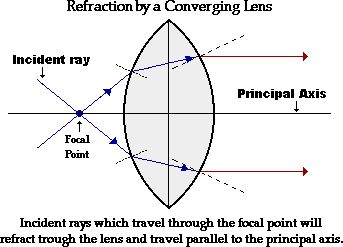# Rules for Convex Lenses

## There are three simple rules that govern how light passes through a thin convex lens.

#### 1. Any incident ray traveling parallel to the principal axis of a converging lens will refract through the lens and travel through the focal point on the opposite side of the lens.#### 2. Any incident ray traveling through the focal point on the way to the lens will refract through the lens and travel parallel to the principal axis.# Ray Diagrams for Convex Lenses

## Now that we understand these basic rules governing lenses we can create what are called ray diagrams in order to predict the position, orientation, and size of the image generated by the lens.

#### 3. The image point of the top of the object is the point where the two refracted rays intersect. Note that if the two refracted rays intersect on the same side of the lens as the object the image formed in called a virtual image.Go to interactive calculator.

# Rules for Concave Lenses

## There are also three simple rules that govern how light passes through a thin concave lens.

#### 1. Any incident ray traveling parallel to the principal axis of a diverging lens will refract through the lens and travel in line with the focal point (i.e., in a direction such that its extension will pass through the focal point).#### 2. Any incident ray traveling towards the focal point on the way to the lens will refract through the lens and travel parallel to the principal axis.# Ray Diagrams for Concave Lenses

## Now that we understand these basic rules governing lenses we can create what are called ray diagrams in order to predict the position, orientation, and size of the image generated by the lens.

#### 3. The image point of the top of the object is the point where the two refracted rays intersect. Since the refracted rays are diverging, they must be extended behind the lens in order to intersect. When the image is formed on the same side of the lens as the object it is know as a virtual image.Go to interactive calculator.